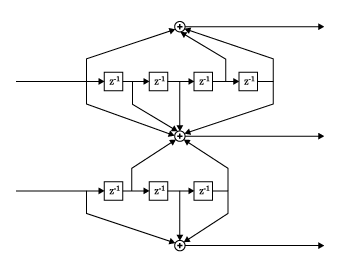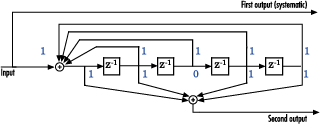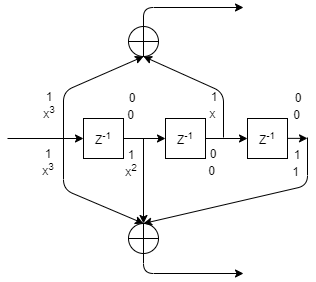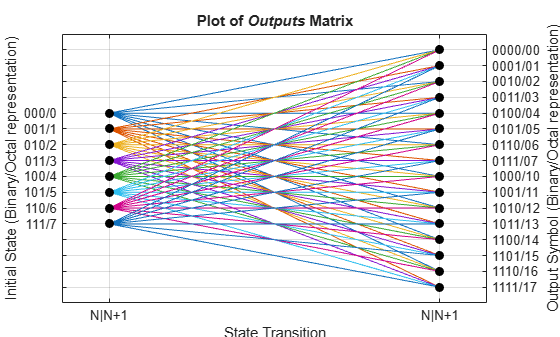# poly2trellis

Convert convolutional code polynomials to trellis description

## Syntax

``trellis = poly2trellis(ConstraintLength,CodeGenerator)``
``trellis = poly2trellis(ConstraintLength,CodeGenerator,FeedbackConnection)``

## Description

example

````trellis = poly2trellis(ConstraintLength,CodeGenerator)` returns the trellis structure description corresponding to the conversion for a rate K / N feedforward encoder. K is the number of input bit streams to the encoder, and N is the number of output connections. `ConstraintLength` specifies the delay for the input bit streams to the encoder. `CodeGenerator` specifies the output connections for the input bit streams to the encoder.The poly2trellis function accepts a polynomial description of a convolutional encoder and returns the corresponding trellis structure description. This output can be used as an input to the `convenc` and `vitdec` functions. It can also be used as a mask parameter value for the Convolutional Encoder, Viterbi Decoder, and APP Decoder blocks. NoteWhen used with a feedback polynomial, poly2trellis makes a feedback connection to the input of the trellis. ```
````trellis = poly2trellis(ConstraintLength,CodeGenerator,FeedbackConnection)` returns the trellis structure description corresponding to the conversion for a rate K / N feedback encoder. K is the number of input bit streams to the encoder, and N is the number of output connections. `ConstraintLength` specifies the delay for the input bit streams to the encoder. `CodeGenerator` specifies the output connections for the input bit streams to the encoder. `FeedbackConnection` specifies the feedback connection for each of the K input bit streams to the encoder.```

## Examples

collapse all

Use a trellis structure to configure the rate 1/2 feedforward convolutional code in this diagram.Create a trellis structure, setting the constraint length to 3 and specifying the code generator as a vector of octal values. The diagram indicates the binary values and polynomial form, indicating the left-most bit is the most-significant-bit (MSB). The binary vector [1 1 0] represents octal 6 and corresponds to the upper row of binary digits in the diagram. The binary vector [1 1 1] represents octal 7 and corresponds to the lower row of binary digits in the diagram. These binary digits indicate connections from the outputs of the registers to the two adders in the diagram.

`trellis = poly2trellis(3,[6 7])`
```trellis = struct with fields: numInputSymbols: 2 numOutputSymbols: 4 numStates: 4 nextStates: [4x2 double] outputs: [4x2 double] ```

Generate random binary data. Convolutionally encode the data, by using the specified trellis structure. Decode the coded data by using the Viterbi algorithm with the specified trellis structure, 34 for its traceback depth, truncated operation mode, and hard decisions.

```data = randi([0 1],70,1); codedData = convenc(data,trellis); tbdepth = 34; decodedData = vitdec(codedData,trellis,tbdepth,'trunc','hard');```

Verify the decoded data has zero bit errors.

`biterr(data,decodedData)`
```ans = 0 ```

Create a trellis structure for a rate 2/3 feedforward convolutional code and display a portion of the next states of the trellis. See `convenc` for an example using this encoder.

The diagram shows a rate 2/3 encoder with two input streams, three output streams, and seven shift registers.Create a trellis structure. Set the constraint length of the upper path to 5 and the constraint length of the lower path to 4. The octal representation of the code generator matrix corresponds to the taps from the upper and lower shift registers.

`trellis = poly2trellis([5 4],[23 35 0; 0 5 13])`
```trellis = struct with fields: numInputSymbols: 4 numOutputSymbols: 8 numStates: 128 nextStates: [128x4 double] outputs: [128x4 double] ```

The structure field `numInputSymbols` equals 4 because two bit streams can produce four different input symbols. The structure field `numOutputSymbols` equals 8 because three bit streams produce eight different output symbols. Because the encoder has seven total shift registers, the number of possible states is ${2}^{7}=128$, as shown by the `nextStates` field.

Display the first five rows of the 128-by-4 `trellis.nextStates` matrix.

`trellis.nextStates(1:5,:)`
```ans = 5×4 0 64 8 72 0 64 8 72 1 65 9 73 1 65 9 73 2 66 10 74 ```

Create a trellis structure to represent the rate 1/2 systematic convolutional encoder with feedback shown in this diagram.This encoder has 5 for its constraint length, [37 33] as its generator polynomial matrix, and 37 for its feedback connection polynomial.

The first generator polynomial is octal 37. The second generator polynomial is octal 33. The feedback polynomial is octal 37. The first generator polynomial matches the feedback connection polynomial because the first output corresponds to the systematic bits.

The binary vector [1 1 1 1 1] represents octal 37 and corresponds to the upper row of binary digits in the diagram. The binary vector [1 1 0 1 1] represents octal 33 and corresponds to the lower row of binary digits in the diagram. These binary digits indicate connections from the outputs of the registers to the two adders in the diagram. The initial 1 corresponds to the input bit.

Convert the polynomial to a trellis structure by using the `poly2trellis` function. When used with a feedback polynomial, `poly2trellis` makes a feedback connection to the input of the trellis.

`trellis = poly2trellis(5,[37 33],37)`
```trellis = struct with fields: numInputSymbols: 2 numOutputSymbols: 4 numStates: 16 nextStates: [16x2 double] outputs: [16x2 double] ```

Generate random binary data. Convolutionally encode the data by using the specified trellis structure. Decode the coded data by using the Viterbi algorithm with the specified trellis structure, 34 for its traceback depth, truncated operation mode, and hard decisions.

```data = randi([0 1],70,1); codedData = convenc(data,trellis); tbdepth = 34; % Traceback depth for Viterbi decoder decodedData = vitdec(codedData,trellis,tbdepth,'trunc','hard');```

Verify the decoded data has zero bit errors.

`biterr(data,decodedData)`
```ans = 0 ```

Demonstrate alternative forms of specifying code generators for a trellis structure are equivalent.Use a trellis structure to configure the rate 1/2 feedforward convolutional code in this diagram. The diagram indicates the binary values and polynomial form, indicating the left-most bit is the most-significant-bit (MSB).

Set the constraint length to 4. Use a cell array of polynomial character vectors to specify code generators. For more information, see Character Representation of Polynomials. When using character representation to specify the code generator, you can specify the polynomial in ascending or descending order, but the `poly2trellis` function always assigns registers in descending order with the left-most register for the MSB.

`trellis_poly = poly2trellis(4,{'x3 + x','x3 + x2 + 1'})`
```trellis_poly = struct with fields: numInputSymbols: 2 numOutputSymbols: 4 numStates: 8 nextStates: [8x2 double] outputs: [8x2 double] ```

The binary vector [1 0 1 0] represents octal 12 and corresponds to the upper row of binary digits in the diagram. The binary vector [1 1 0 1] represents octal 15 and corresponds to the lower row of binary digits in the diagram. Use octal representation to specify the code generators for an equivalent trellis structure.

`trellis = poly2trellis(4,[12 15])`
```trellis = struct with fields: numInputSymbols: 2 numOutputSymbols: 4 numStates: 8 nextStates: [8x2 double] outputs: [8x2 double] ```

Use `isequal` to confirm the two trellises are equal.

`isequal(trellis,trellis_poly)`
```ans = logical 1 ```

This example demonstrates creation of a nonstandard trellis structure for a convolutional encoder with uncoded bits and feedback. The encoder cannot be created using `poly2trellis` because the peculiar specifications for the encoder do not match the input requirements of `poly2trellis`.

You can manually create the trellis structure, and then use it as the input trellis structure for an encoder and decoder. The Convolutional Encoder and Viterbi Decoder blocks used in the Convolutional Encoder with Uncoded Bits and Feedback model load the trellis structure created here using a `PreLoadFcn` callback.

Convolutional Encoder

Create a rate 3/4 convolutional encoder with feedback connection whose MSB bit remains uncoded.Declare variables according to the specifications.

```K = 3; N = 4; constraintLength = 4; ```

Create trellis structure

A trellis is represented by a structure with the following fields:

• `numInputSymbols` – Number of input symbols

• `numOutputSymbols` – Number of output symbols

• `numStates` – Number of states

• `nextStates` – Next state matrix

• `outputs` – Output matrix

For more information about these structure fields, see `istrellis`.

Reset any previous occurrence of `myTrellis` structure.

`clear myTrellis;`

Define the trellis structure fields.

```myTrellis.numInputSymbols = 2^K; myTrellis.numOutputSymbols = 2^N; myTrellis.numStates = 2^(constraintLength-1);```

Create `nextStates` Matrix

The `nextStates` matrix is a [`numStates` x `numInputSymbols`] matrix. The (i,j) element of the next state matrix is the resulting final state index that corresponds to a transition from the initial state i for an input equal to j.

```myTrellis.nextStates = [0 1 2 3 0 1 2 3; ... 6 7 4 5 6 7 4 5; ... 1 0 3 2 1 0 3 2; ... 7 6 5 4 7 6 5 4; ... 2 3 0 1 2 3 0 1; ... 4 5 6 7 4 5 6 7; ... 3 2 1 0 3 2 1 0; ... 5 4 7 6 5 4 7 6] ```
```myTrellis = struct with fields: numInputSymbols: 8 numOutputSymbols: 16 numStates: 8 nextStates: [8x8 double] ```

Plot `nextStates` Matrix

Use the `commcnv_plotnextstates` helper function to plot the `nextStates` matrix to illustrate the branch transitions between different states for a given input.

`commcnv_plotnextstates(myTrellis.nextStates);`Create` outputs `Matrix

The `outputs` matrix is a [`numStates` x `numInputSymbols`] matrix. The (i,j) element of the output matrix is the output symbol in octal format given a current state i for an input equal to j.

```outputs = [0 2 4 6 10 12 14 16; ... 1 3 5 7 11 13 15 17; ... 0 2 4 6 10 12 14 16; ... 1 3 5 7 11 13 15 17; ... 0 2 4 6 10 12 14 16; ... 1 3 5 7 11 13 15 17; ... 0 2 4 6 10 12 14 16; ... 1 3 5 7 11 13 15 17]```
```outputs = 8×8 0 2 4 6 10 12 14 16 1 3 5 7 11 13 15 17 0 2 4 6 10 12 14 16 1 3 5 7 11 13 15 17 0 2 4 6 10 12 14 16 1 3 5 7 11 13 15 17 0 2 4 6 10 12 14 16 1 3 5 7 11 13 15 17 ```

Use `oct2dec` to display these values in decimal format.

`outputs_dec = oct2dec(outputs)`
```outputs_dec = 8×8 0 2 4 6 8 10 12 14 1 3 5 7 9 11 13 15 0 2 4 6 8 10 12 14 1 3 5 7 9 11 13 15 0 2 4 6 8 10 12 14 1 3 5 7 9 11 13 15 0 2 4 6 8 10 12 14 1 3 5 7 9 11 13 15 ```

Copy outputs matrix into the `myTrellis` structure.

`myTrellis.outputs = outputs`
```myTrellis = struct with fields: numInputSymbols: 8 numOutputSymbols: 16 numStates: 8 nextStates: [8x8 double] outputs: [8x8 double] ```

Plot `outputs` Matrix

Use the `commcnv_plotoutputs` helper function to plot the `outputs` matrix to illustrate the possible output symbols for a given state depending on the input symbol.

`commcnv_plotoutputs(myTrellis.outputs, myTrellis.numOutputSymbols);`Check Resulting Trellis Structure

`istrellis(myTrellis)`
```ans = logical 1 ```

A return value of `1` confirms the trellis structure is valid.

Decode with 3-bit soft decisions partitioned so that values near 0 map to 0, and values near 1 map to 7. If your application requires better decoding performance, refine the partition to obtain finer quantization.

The example decodes the code and computes the bit error rate. When comparing the decoded data with the original message, the example must take the decoding delay into account. The continuous operation mode of the Viterbi decoder causes a delay equal to the traceback length, so `msg(1)` corresponds to `decoded(tblen+1)` rather than to `decoded(1)`.

System Setup

Initialize runtime variables for the message data, trellis, bit error rate computations, and traceback length.

```stream = RandStream.create('mt19937ar', 'seed',94384); prevStream = RandStream.setGlobalStream(stream); msg = randi([0 1],4000,1); % Random data trellis = poly2trellis(7,[171 133]); % Define trellis ber = zeros(3,1); % Store BER values tblen = 48; % Traceback length```

Create an AWGN channel System object, a Viterbi decoder System object, and an error rate calculator System object. Account for the receive delay caused by the traceback length of the Viterbi decoder.

```awgnChan = comm.AWGNChannel('NoiseMethod','Signal to noise ratio (SNR)','SNR',6); vitDec = comm.ViterbiDecoder(trellis,'InputFormat','Soft', ... 'SoftInputWordLength',3,'TracebackDepth',tblen,'TerminationMethod','Continuous'); errorCalc = comm.ErrorRate('ReceiveDelay', tblen);```

Run Coding and Decoding

Convolutionally code the message, pass in through an AWGN filter, quantize the noisy message for soft-decision decoding. Perform Viterbi decoding using the trellis generated using `poly2trellis`.

```code = convenc(msg,trellis); awgnChan.SignalPower = (code'*code)/length(code); ncode = awgnChan(code);```

Use `quantiz` to map the noisy data values to appropriate decision-value integers between 0 and 7. The second argument in `quantiz` is a partition vector that determines which data values map to 0, 1, 2, etc.

```qcode = quantiz(ncode,[0.001,0.1,0.3,0.5,0.7,0.9,0.999]); decoded = vitDec(qcode);```

Compute bit error rate.

```ber = errorCalc(msg,decoded); ratio = ber(1)```
```ratio = 0.0013 ```
`number = ber(2)`
```number = 5 ```
`RandStream.setGlobalStream(prevStream);`

## Input Arguments

collapse all

Constraint length, specified as a 1-by-K row vector defining the delay for each of the K input bit streams to the encoder.

Data Types: `double`

Code generator, specified as a K-by-N matrix of octal numbers, a K-by-N cell array of polynomial character vectors, or a K-by-N string array. `CodeGenerator` specifies the N output connections for each of the K input bit streams to the encoder.

When using character representation to specify the code generator, you can specify the polynomial in ascending or descending order, but the `poly2trellis` function always assigns registers in descending order with the left-most register for the most-significant-bit (MSB). For more information, see Specifying Code Generators in Polynomial Form.

Data Types: `double` | `char` | `string`

Feedback connection, specified as a 1-by-K row vector of octal numbers defining the feedback connection for each of the K input bit streams to the encoder.

Data Types: `double`

## Output Arguments

collapse all

Trellis description, returned as a structure with these fields. For more about this structure, see the `istrellis` function.

Trellis Structure Fields for Rate K/N Code

Number of input symbols, returned as a scalar with a value of 2K. This value represents the number of input symbols to the encoder and K represents the number of input bit streams.

Number of output symbols, returned as a scalar with a value of 2N. This value represents the number of output symbols from the encoder and N represents the number of output bit streams.

Number of states in the encoder, returned as a scalar.

Next states for all combinations of current states and current inputs, returned as a `numStates`-by-2K matrix, where K represents the number of input bit streams.

Outputs for all combinations of current states and current inputs, returned as a `numStates`-by-2K matrix, K represents the number of input bit streams. The elements of this matrix are octal numbers.

## Extended Capabilities

### Topics

Introduced before R2006a